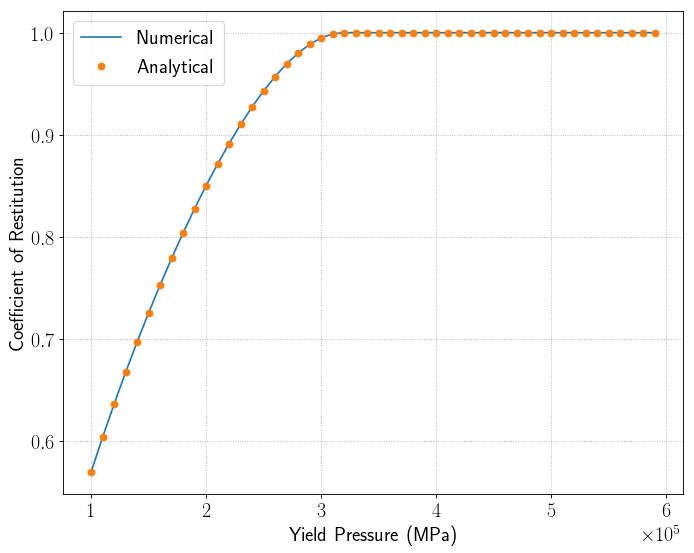# Numerical analysis of an elasto-plastic model¶

In this tutorial, we compute the coefficient of restitution of the Thornton-Ning contact model over a range of yielding pressures. The input script file is found in examples/scripts/Contact-Model/elasto-plastic.

In :
# Import simulation module
from PyGran import simulation as sim

# Import an organic (cohesionless) powder
from PyGran.params import cohesionless

# Import numpy module
from numpy import arange, fabs, sqrt, array

# Contact model
cModel = sim.models.ThorntonNing

# Create a list to store the coefs of restitution
COR, yieldVel = [], []

# Create a yield pressure array to study
pressure = arange(1, 6, 0.1) * cohesionless['youngsModulus'] * 0.01

# Set particle radius to 100 microns
cohesionless['radius'] = 1e-4

for yieldPress in pressure:

cohesionless['yieldPress'] = yieldPress
model = cModel(material=cohesionless)

time, disp, force = model.displacement()
deltav = disp[:,1]

COR.append(fabs(deltav[-1] / cohesionless['characteristicVelocity']))
yieldVel.append( model.yieldVel / cohesionless['characteristicVelocity'] )

Out: